• matlab建立矩阵
千次阅读
2021-04-18 03:42:10

查看原文：http://houyewei.com/archives/493

2.2.1++矩阵的建立

1．直接输入法

++++最简单的建立矩阵的方法是从键盘直接输入矩阵的元素。具体方法如下：将矩阵的元素用方括号括起来，按矩阵行的顺序输入各元素，同一行的各元素之间用空格或逗号分隔，不同行的元素之间用分号分隔。

+

2．利用M文件建立矩阵

++++对于比较大且比较复杂的矩阵，可以为它专门建立一个M文件。下面通过一个简单例子来说明如何利用M文件创建矩阵。

+

++++例2-2++利用M文件建立MYMAT矩阵。

(1)+启动有关编辑程序或MATLAB文本编辑器，并输入待建矩阵：

(2)+把输入的内容以纯文本方式存盘(设文件名为mymatrix.m)。

(3)+在MATLAB命令窗口中输入mymatrix，即运行该M文件，就会自动建立一个名为MYMAT的矩阵，可供以后使用。

+

3．利用冒号表达式建立一个向量

++++冒号表达式可以产生一个行向量，一般格式是：

++++e1:e2:e3

其中e1为初始值，e2为步长，e3为终止值。

在MATLAB中，还可以用linspace函数产生行向量。其调用格式为：

linspace(a,b,n)

其中a和b是生成向量的第一个和最后一个元素，n是元素总数。

显然，linspace(a,b,n)与a:(b-a)/(n-1):b等价。

4．建立大矩阵

大矩阵可由方括号中的小矩阵或向量建立起来。

+

2.2.2++矩阵的拆分

1．矩阵元素

++++通过下标引用矩阵的元素，例如

A(3,2)=200

采用矩阵元素的序号来引用矩阵元素。矩阵元素的序号就是相应元素在内存中的排列顺序。在MATLAB中，矩阵元素按列存储，先第一列，再第二列，依次类推。例如

A=[1,2,3;4,5,6];

A(3)

ans+=

+++++2

显然，序号(Index)与下标(Subscript+)是一一对应的，以m×n矩阵A为例，矩阵元素A(i,j)的序号为(j-1)*m+i。其相互转换关系也可利用sub2ind和ind2sub函数求得。

+

2．矩阵拆分

++++(1)+利用冒号表达式获得子矩阵

++++++++①+A(:,j)表示取A矩阵的第j列全部元素；A(i,:)表示A矩阵第i行的全部元素；A(i,j)表示取A矩阵第i行、第j列的元素。

++++++++②+A(i:i+m,:)表示取A矩阵第i～i+m行的全部元素；A(:,k:k+m)表示取A矩阵第k～k+m列的全部元素，A(i:i+m,k:k+m)表示取A矩阵第i～i+m行内，并在第k～k+m列中的所有元素。

此外，还可利用一般向量和end运算符来表示矩阵下标，从而获得子矩阵。end表示某一维的末尾元素下标。

+

++++(2)+利用空矩阵删除矩阵的元素

++++在MATLAB中，定义[]为空矩阵。给变量X赋空矩阵的语句为X=[]。注意，X=[]与clear+X不同，clear是将X从工作空间中删除，而空矩阵则存在于工作空间中，只是维数为0。

+

2.2.3++特殊矩阵

1．通用的特殊矩阵

常用的产生通用特殊矩阵的函数有：

zeros：产生全0矩阵(零矩阵)。

ones：产生全1矩阵(幺矩阵)。

eye：产生单位矩阵。

rand：产生0～1间均匀分布的随机矩阵。

randn：产生均值为0，方差为1的标准正态分布随机矩阵。

+

例2-3++分别建立3×3、3×2和与矩阵A同样大小的零矩阵。

(1)+建立一个3×3零矩阵。

zeros(3)

(2)+建立一个3×2零矩阵。

zeros(3,2)

(3)+设A为2×3矩阵，则可以用zeros(size(A))建立一个与矩阵A同样大小零矩阵。

A=[1+2+3;4+5+6];++++%产生一个2×3阶矩阵A

zeros(size(A))++++++%产生一个与矩阵A同样大小的零矩阵

+

例2-4+建立随机矩阵：

(1)+在区间[20,50]内均匀分布的5阶随机矩阵。

(2)+均值为0.6、方差为0.1的5阶正态分布随机矩阵。

命令如下：

x=20+(50-20)*rand(5)

y=0.6+sqrt(0.1)*randn(5)

此外，常用的函数还有reshape(A,m,n)，它在矩阵总元素保持不变的前提下，将矩阵A重新排成m×n的二维矩阵。

+

2．用于专门学科的特殊矩阵

(1)+魔方矩阵

魔方矩阵有一个有趣的性质，其每行、每列及两条对角线上的元素和都相等。对于n阶魔方阵，其元素由1,2,3,…,n2共n2个整数组成。MATLAB提供了求魔方矩阵的函数magic(n)，其功能是生成一个n阶魔方阵。

+

例2-5++将101~125等25个数填入一个5行5列的表格中，使其每行每列及对角线的和均为565。

M=100+magic(5)

+

(2)+范得蒙矩阵

范得蒙(Vandermonde)矩阵最后一列全为1，倒数第二列为一个指定的向量，其他各列是其后列与倒数第二列的点乘积。可以用一个指定向量生成一个范得蒙矩阵。在MATLAB中，函数vander(V)生成以向量V为基础向量的范得蒙矩阵。例如，A=vander([1;2;3;5])即可得到上述范得蒙矩阵。

(3)+希尔伯特矩阵

在MATLAB中，生成希尔伯特矩阵的函数是hilb(n)。

使用一般方法求逆会因为原始数据的微小扰动而产生不可靠的计算结果。MATLAB中，有一个专门求希尔伯特矩阵的逆的函数invhilb(n)，其功能是求n阶的希尔伯特矩阵的逆矩阵。

+

例2-6++求4阶希尔伯特矩阵及其逆矩阵。

命令如下：

format+rat+++++%以有理形式输出

H=hilb(4)

H=invhilb(4)

(4)+托普利兹矩阵

托普利兹(Toeplitz)矩阵除第一行第一列外，其他每个元素都与左上角的元素相同。生成托普利兹矩阵的函数是toeplitz(x,y)，它生成一个以x为第一列，y为第一行的托普利兹矩阵。这里x,+y均为向量，两者不必等长。toeplitz(x)用向量x生成一个对称的托普利兹矩阵。例如

T=toeplitz(1:6)

+

(5)+伴随矩阵

MATLAB生成伴随矩阵的函数是compan(p)，其中p是一个多项式的系数向量，高次幂系数排在前，低次幂排在后。例如，为了求多项式的x3-7x+6的伴随矩阵，可使用命令：

p=[1,0,-7,6];

compan(p)

+

(6)+帕斯卡矩阵

我们知道，二次项(x+y)n展开后的系数随n的增大组成一个三角形表，称为杨辉三角形。由杨辉三角形表组成的矩阵称为帕斯卡(Pascal)矩阵。函数pascal(n)生成一个n阶帕斯卡矩阵。

+

例2-7++求(x+y)5的展开式。

在MATLAB命令窗口，输入命令：

pascal(6)

矩阵次对角线上的元素1,5,10,10,5,1即为展开式的系数。

+

2.3++MATLAB运算+

2.3.1算术运算

1．基本算术运算

++++MATLAB的基本算术运算有：＋(加)、－(减)、*(乘)、/(右除)、(左除)、^(乘方)。

注意，运算是在矩阵意义下进行的，单个数据的算术运算只是一种特例。

+

++++(1)+矩阵加减运算

++++假定有两个矩阵A和B，则可以由A+B和A-B实现矩阵的加减运算。运算规则是：若A和B矩阵的维数相同，则可以执行矩阵的加减运算，A和B矩阵的相应元素相加减。如果A与B的维数不相同，则MATLAB将给出错误信息，提示用户两个矩阵的维数不匹配。

+

++++(2)+矩阵乘法++

假定有两个矩阵A和B，若A为m×n矩阵，B为n×p矩阵，则C=A*B为m×p矩阵。

+

++++(3)+矩阵除法

在MATLAB中，有两种矩阵除法运算：和/，分别表示左除和右除。如果A矩阵是非奇异方阵，则AB和B/A运算可以实现。AB等效于A的逆左乘B矩阵，也就是inv(A)*B，而B/A等效于A矩阵的逆右乘B矩阵，也就是B*inv(A)。

对于含有标量的运算，两种除法运算的结果相同，如3/4和43有相同的值，都等于0.75。又如，设a=[10.5,25]，则a/5=5a=[2.1000+5.0000]。对于矩阵来说，左除和右除表示两种不同的除数矩阵和被除数矩阵的关系。对于矩阵运算，一般AB≠B/A。

+

++++(4)+矩阵的乘方

++++一个矩阵的乘方运算可以表示成A^x，要求A为方阵，x为标量。

2．点运算

++++在MATLAB中，有一种特殊的运算，因为其运算符是在有关算术运算符前面加点，所以叫点运算。点运算符有.*、./、.和.^。两矩阵进行点运算是指它们的对应元素进行相关运算，要求两矩阵的维参数相同。

+

2.3.2++关系运算

++++MATLAB提供了6种关系运算符：(大于)、>=(大于或等于)、==(等于)、～=(不等于)。它们的含义不难理解，但要注意其书写方法与数学中的不等式符号不尽相同。

+

++++关系运算符的运算法则为：

++++(1)+当两个比较量是标量时，直接比较两数的大小。若关系成立，关系表达式结果为1，否则为0。

++++(2)+当参与比较的量是两个维数相同的矩阵时，比较是对两矩阵相同位置的元素按标量关系运算规则逐个进行，并给出元素比较结果。最终的关系运算的结果是一个维数与原矩阵相同的矩阵，它的元素由0或1组成。

+

++++(3)+当参与比较的一个是标量，而另一个是矩阵时，则把标量与矩阵的每一个元素按标量关系运算规则逐个比较，并给出元素比较结果。最终的关系运算的结果是一个维数与原矩阵相同的矩阵，它的元素由0或1组成。

+

++++例2-8++产生5阶随机方阵A，其元素为[10,90]区间的随机整数，然后判断A的元素是否能被3整除。

++++(1)+生成5阶随机方阵A。

A=fix((90-10+1)*rand(5)+10)

++++(2)+判断A的元素是否可以被3整除。

+P=rem(A,3)==0

其中，rem(A,3)是矩阵A的每个元素除以3的余数矩阵。此时，0被扩展为与A同维数的零矩阵，P是进行等于(==)比较的结果矩阵。

+

2.3.3++逻辑运算

++++MATLAB提供了3种逻辑运算符：&(与)、|(或)和～(非)。

++++逻辑运算的运算法则为：

(1)+在逻辑运算中，确认非零元素为真，用1表示，零元素为假，用0表示。

(2)+设参与逻辑运算的是两个标量a和b，那么，

++++a&b++a,b全为非零时，运算结果为1，否则为0。

++++a|b++a,b中只要有一个非零，运算结果为1。

++++～a++当a是零时，运算结果为1；当a非零时，运算结果为0。

+

(3)+若参与逻辑运算的是两个同维矩阵，那么运算将对矩阵相同位置上的元素按标量规则逐个进行。最终运算结果是一个与原矩阵同维的矩阵，其元素由1或0组成。

(4)+若参与逻辑运算的一个是标量，一个是矩阵，那么运算将在标量与矩阵中的每个元素之间按标量规则逐个进行。最终运算结果是一个与矩阵同维的矩阵，其元素由1或0组成。

(5)+逻辑非是单目运算符，也服从矩阵运算规则。

(6)+在算术、关系、逻辑运算中，算术运算优先级最高，逻辑运算优先级最低。

+

例2-9++建立矩阵A，然后找出大于4的元素的位置。

(1)+建立矩阵A。

A=[4,-65,-54,0,6;56,0,67,-45,0]

(2)+找出大于4的元素的位置。

find(A>4)

+

2.4+矩阵分析

2.4.1++对角阵与三角阵

1．对角阵

只有对角线上有非0元素的矩阵称为对角矩阵，对角线上的元素相等的对角矩阵称为数量矩阵，对角线上的元素都为1的对角矩阵称为单位矩阵。

+

(1)+提取矩阵的对角线元素

设A为m×n矩阵，diag(A)函数用于提取矩阵A主对角线元素，产生一个具有min(m,n)个元素的列向量。

diag(A)函数还有一种形式diag(A,k)，其功能是提取第k条对角线的元素。

(2)+构造对角矩阵

设V为具有m个元素的向量，diag(V)将产生一个m×m对角矩阵，其主对角线元素即为向量V的元素。

diag(V)函数也有另一种形式diag(V,k)，其功能是产生一个n×n(n=m+)对角阵，其第k条对角线的元素即为向量V的元素。

+

例2-10++先建立5×5矩阵A，然后将A的第一行元素乘以1，第二行乘以2，…，第五行乘以5。

A=[17,0,1,0,15;23,5,7,14,16;4,0,13,0,22;10,12,19,21,3;...

11,18,25,2,19];

D=diag(1:5);

D*A++++++++++++++++%用D左乘A，对A的每行乘以一个指定常数

+

2．三角阵

三角阵又进一步分为上三角阵和下三角阵，所谓上三角阵，即矩阵的对角线以下的元素全为0的一种矩阵，而下三角阵则是对角线以上的元素全为0的一种矩阵。

+

(1)+上三角矩阵

求矩阵A的上三角阵的MATLAB函数是triu(A)。

triu(A)函数也有另一种形式triu(A,k)，其功能是求矩阵A的第k条对角线以上的元素。例如，提取矩阵A的第2条对角线以上的元素，形成新的矩阵B。

(2)+下三角矩阵

在MATLAB中，提取矩阵A的下三角矩阵的函数是tril(A)和tril(A,k)，其用法与提取上三角矩阵的函数triu(A)和triu(A,k)完全相同。

+

2.4.2++矩阵的转置与旋转

1．矩阵的转置

转置运算符是单撇号(‘)。

2．矩阵的旋转

利用函数rot90(A,k)将矩阵A旋转90º的k倍，当k为1时可省略。

3．矩阵的左右翻转

对矩阵实施左右翻转是将原矩阵的第一列和最后一列调换，第二列和倒数第二列调换，…，依次类推。MATLAB对矩阵A实施左右翻转的函数是fliplr(A)。

4．矩阵的上下翻转

MATLAB对矩阵A实施上下翻转的函数是flipud(A)。

+

2.4.3++矩阵的逆与伪逆

1．矩阵的逆

对于一个方阵A，如果存在一个与其同阶的方阵B，使得：

A•B=B•A=I+(I为单位矩阵)

则称B为A的逆矩阵，当然，A也是B的逆矩阵。

求一个矩阵的逆是一件非常烦琐的工作，容易出错，但在MATLAB中，求一个矩阵的逆非常容易。求方阵A的逆矩阵可调用函数inv(A)。

例2-11++用求逆矩阵的方法解线性方程组。

Ax=b

其解为：

x=A-1b

+

2．矩阵的伪逆

如果矩阵A不是一个方阵，或者A是一个非满秩的方阵时，矩阵A没有逆矩阵，但可以找到一个与A的转置矩阵A‘同型的矩阵B，使得：

A•B•A=A

B•A•B=B

此时称矩阵B为矩阵A的伪逆，也称为广义逆矩阵。在MATLAB中，求一个矩阵伪逆的函数是pinv(A)。

+

2.4.4+方阵的行列式

把一个方阵看作一个行列式，并对其按行列式的规则求值，这个值就称为矩阵所对应的行列式的值。在MATLAB中，求方阵A所对应的行列式的值的函数是det(A)。

+

2.4.5++矩阵的秩与迹

1．矩阵的秩

矩阵线性无关的行数与列数称为矩阵的秩。在MATLAB中，求矩阵秩的函数是rank(A)。

2．矩阵的迹

矩阵的迹等于矩阵的对角线元素之和，也等于矩阵的特征值之和。在MATLAB中，求矩阵的迹的函数是trace(A)。

+

2.4.6++向量和矩阵的范数

矩阵或向量的范数用来度量矩阵或向量在某种意义下的长度。范数有多种方法定义，其定义不同，范数值也就不同。

+

1．向量的3种常用范数及其计算函数

在MATLAB中，求向量范数的函数为：

(1)+norm(V)或norm(V,2)：计算向量V的2—范数。

(2)+norm(V,1)：计算向量V的1—范数。

(3)+norm(V,inf)：计算向量V的∞—范数。

2．矩阵的范数及其计算函数

MATLAB提供了求3种矩阵范数的函数，其函数调用格式与求向量的范数的函数完全相同。

2.4.7++矩阵的条件数

在MATLAB中，计算矩阵A的3种条件数的函数是：

(1)+cond(A,1)+++计算A的1—范数下的条件数。

(2)+cond(A)或cond(A,2)+++计算A的2—范数数下的条件数。

(3)+cond(A,inf)+++计算A的+∞—范数下的条件数。

+

2.4.8++矩阵的特征值与特征向量

在MATLAB中，计算矩阵A的特征值和特征向量的函数是eig(A)，常用的调用格式有3种：

(1)+E=eig(A)：求矩阵A的全部特征值，构成向量E。

(2)+[V,D]=eig(A)：求矩阵A的全部特征值，构成对角阵D，并求A的特征向量构成V的列向量。

(3)+[V,D]=eig(A,‘nobalance’)：与第2种格式类似，但第2种格式中先对A作相似变换后求矩阵A的特征值和特征向量，而格式3直接求矩阵A的特征值和特征向量。

+

例2-12++用求特征值的方法解方程。

3x5-7x4+5x2+2x-18=0

p=[3,-7,0,5,2,-18];

A=compan(p);+++++++++++%A的伴随矩阵

x1=eig(A)++++++++++++++%求A的特征值

x2=roots(p)+++++++++++++++++%直接求多项式p的零点

+

2.5+矩阵的超越函数

1．矩阵平方根sqrtm

sqrtm(A)计算矩阵A的平方根。

2．矩阵对数logm

logm(A)计算矩阵A的自然对数。此函数输入参数的条件与输出结果间的关系和函数sqrtm(A)完全一样

3．矩阵指数expm、expm1、expm2、expm3

expm(A)、expm1(A)、expm2(A)、expm3(A)的功能都求矩阵指数eA。

4．普通矩阵函数funm

funm(A,‘fun’)用来计算直接作用于矩阵A的由‘fun’指定的超越函数值。当fun取sqrt时，funm(A,‘sqrt’)可以计算矩阵A的平方根，与sqrtm(A)的计算结果一样。

+

2.6++字符串

++++在MATLAB中，字符串是用单撇号括起来的字符序列。

MATLAB将字符串当作一个行向量，每个元素对应一个字符，其标识方法和数值向量相同。也可以建立多行字符串矩阵。

+

字符串是以ASCII码形式存储的。abs和double函数都可以用来获取字符串矩阵所对应的ASCII码数值矩阵。相反，char函数可以把ASCII码矩阵转换为字符串矩阵。

+

例2-13++建立一个字符串向量，然后对该向量做如下处理：

(1)+取第1～5个字符组成的子字符串。

(2)+将字符串倒过来重新排列。

(3)+将字符串中的小写字母变成相应的大写字母，其余字符不变。

(4)+统计字符串中小写字母的个数。

+

命令如下：

ch=‘ABc123d4e56Fg9’;

subch=ch(1:5)+++++++++++%取子字符串

revch=ch(end:-1:1)+++++++%将字符串倒排

k=find(ch>=‘a’&ch

ch(k)=ch(k)-(‘a’-‘A’);+++++++++%将小写字母变成相应的大写字母

char(ch)++++++++++++++++

length(k)++++++++++++++++++%统计小写字母的个数

++++与字符串有关的另一个重要函数是eval，其调用格式为：

++++eval_r(t)

其中t为字符串。它的作用是把字符串的内容作为对应的MATLAB语句来执行。

+

2.7++结构数据和单元数据

2.7.1++结构数据

1．结构矩阵的建立与引用

结构矩阵的元素可以是不同的数据类型，它能将一组具有不同属性的数据纳入到一个统一的变量名下进行管理。建立一个结构矩阵可采用给结构成员赋值的办法。具体格式为：

结构矩阵名.成员名=表达式

其中表达式应理解为矩阵表达式。

+

2．结构成员的修改

可以根据需要增加或删除结构的成员。例如要给结构矩阵a增加一个成员x4，可给a中任意一个元素增加成员x4：

a(1).x4=‘410075’;

但其他成员均为空矩阵，可以使用赋值语句给它赋确定的值。

要删除结构的成员，则可以使用rmfield函数来完成。例如，删除成员x4：

a=rmfield(a,‘x4’);

3．关于结构的函数

除了一般的结构数据的操作外，MATLAB还提供了部分函数来进行结构矩阵的操作。

+

2.7.2++单元数据

1．单元矩阵的建立与引用

建立单元矩阵和一般矩阵相似，只是矩阵元素用大括号括起来。

可以用带有大括号下标的形式引用单元矩阵元素。例如b{3,3}。单元矩阵的元素可以是结构或单元数据。

可以使用celldisp函数来显示整个单元矩阵，如celldisp(b)。还可以删除单元矩阵中的某个元素。2．关于单元的函数

MATLAB还提供了部分函数用于单元的操作。

+

2.8+稀疏矩阵

2.8.1++矩阵存储方式

MATLAB的矩阵有两种存储方式：完全存储方式和稀疏存储方式。

1．完全存储方式

完全存储方式是将矩阵的全部元素按列存储。以前讲到的矩阵的存储方式都是按这个方式存储的，此存储方式对稀疏矩阵也适用。

+

2．稀疏存储方式

稀疏存储方式仅存储矩阵所有的非零元素的值及其位置，即行号和列号。在MATLAB中，稀疏存储方式也是按列存储的。

注意，在讲稀疏矩阵时，有两个不同的概念，一是指矩阵的0元素较多，该矩阵是一个具有稀疏特征的矩阵，二是指采用稀疏方式存储的矩阵。

+

2.8.2++稀疏存储方式的产生

1．将完全存储方式转化为稀疏存储方式

函数A=sparse(S)将矩阵S转化为稀疏存储方式的矩阵A。当矩阵S是稀疏存储方式时，则函数调用相当于A=S。

sparse函数还有其他一些调用格式：

sparse(m,n)：生成一个m×n的所有元素都是0的稀疏矩阵。

sparse(u,v,S)¬¬：u,v,S是3个等长的向量。S是要建立的稀疏矩阵的非0元素，u(i)、v(i)分别是S(i)的行和列下标，该函数建立一个max(u)行、max(v)列并以S为稀疏元素的稀疏矩阵。

此外，还有一些和稀疏矩阵操作有关的函数。例如

[u,v,S]=find(A)：返回矩阵A中非0元素的下标和元素。这里产生的u,v,S可作为sparse(u,v,S)的参数。

full(A)：返回和稀疏存储矩阵A对应的完全存储方式矩阵。

+

2．产生稀疏存储矩阵

只把要建立的稀疏矩阵的非0元素及其所在行和列的位置表示出来后由MATLAB自己产生其稀疏存储，这需要使用spconvert函数。调用格式为：

B=spconvert(A)

其中A为一个m×3或m×4的矩阵，其每行表示一个非0元素，m是非0元素的个数，A每个元素的意义是：

(i,1)++第i个非0元素所在的行。

(i,2)++第i个非0元素所在的列。

(i,3)++第i个非0元素值的实部。

(i,4)++第i个非0元素值的虚部，若矩阵的全部元素都是实数，则无须第四列。

该函数将A所描述的一个稀疏矩阵转化为一个稀疏存储矩阵。

+

例2-15++根据表示稀疏矩阵的矩阵A，产生一个稀疏存储方式矩阵B。

命令如下：

A=[2,2,1;3,1,-1;4,3,3;5,3,8;6,6,12];

B=spconvert(A)

+

3．带状稀疏存储矩阵

用spdiags函数产生带状稀疏矩阵的稀疏存储，调用格式是：

A=spdiags(B,d,m,n)

其中，参数m,n为原带状矩阵的行数与列数。B为r×p阶矩阵，这里r=min(m,n)，p为原带状矩阵所有非零对角线的条数，矩阵B的第i列即为原带状矩阵的第i条非零对角线。

+

4．单位矩阵的稀疏存储

单位矩阵只有对角线元素为1，其他元素都为0，是一种具有稀疏特征的矩阵。函数eye产生一个完全存储方式的单位矩阵。MATLAB还有一个产生稀疏存储方式的单位矩阵的函数，这就是speye。函数speye(m,n)返回一个m×n的稀疏存储单位矩阵。

+

2.8.3++稀疏矩阵应用举例

稀疏存储矩阵只是矩阵的存储方式不同，它的运算规则与普通矩阵是一样的。所以，在运算过程中，稀疏存储矩阵可以直接参与运算。当参与运算的对象不全是稀疏存储矩阵时，所得结果一般是完全存储形式

更多相关内容
• 使用matlab解方程 用解带有变量参数的方程 对数值进行四舍五入的方法 利用矩阵元素不同进行绘图方法 pcolor
• ## 在MATLAB中生成矩阵的三种方法

万次阅读 多人点赞 2021-07-03 10:54:02
MATLAB中生成矩阵的三种方法 1. 直接输入法        1） 矩阵所有元素必须在[]内        2） 矩阵中同行元素以逗号‘,’或空格分隔        3） 矩阵...

# 1. 直接输入法

(1）矩阵所有元素必须在[]内
(2）矩阵中同行元素以逗号‘,’或空格分隔
(3）矩阵中行与行之间用分号‘;’或回车分隔
(4）矩阵元素可以是表达式
(5）若[]中无元素表示空矩阵
同行逗号，行与行分号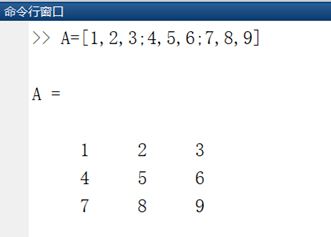同行逗号，行与行回车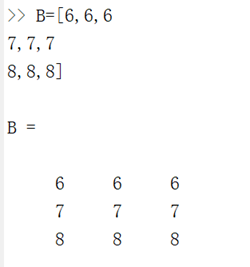同行空格，行与行分号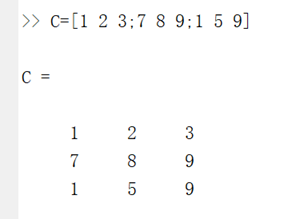同行空格，行与行回车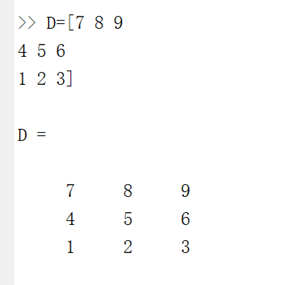# 2. 外部文件读入法

多用于外部大量数据的引入
D盘建如下txt文件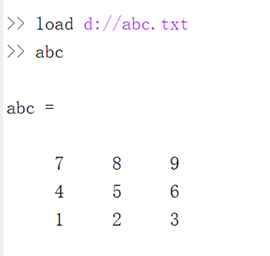2）命令A=import(‘文件名’)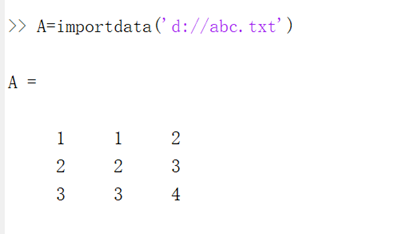从当前程序所在文件夹里，按照函数中指定的法范围，从单元格开始读取文件，返回数据。

# 3.特殊矩阵函数生成法

1）单位矩阵
eye(m,n)
第一个参数代表行数，第二个参数代表列数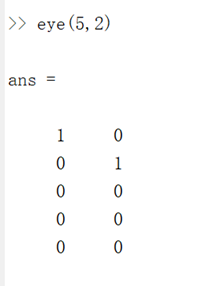eye(m)
若只有一个参数，代表行列相同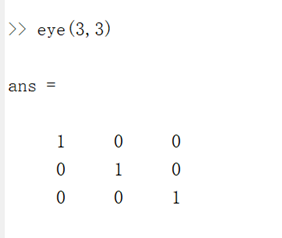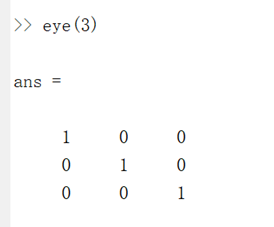2）零矩阵
zeros(m,n)、zeros(m)
与eye类似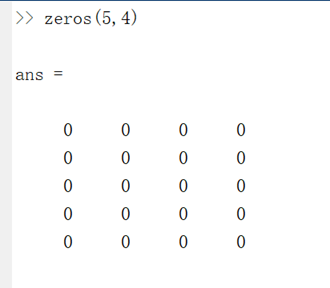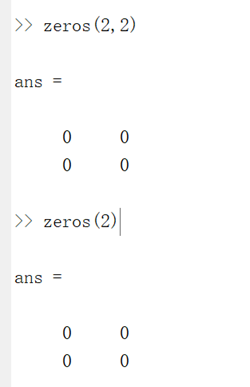3）一矩阵
ones(m,n)、ones(m)同上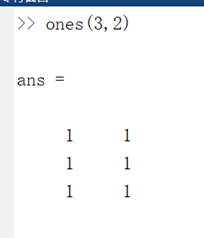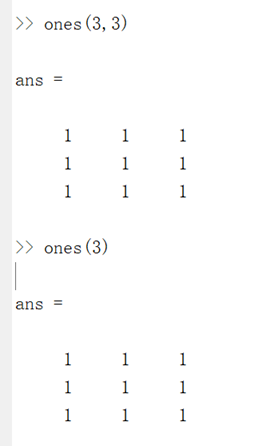4）a中为对角元素向量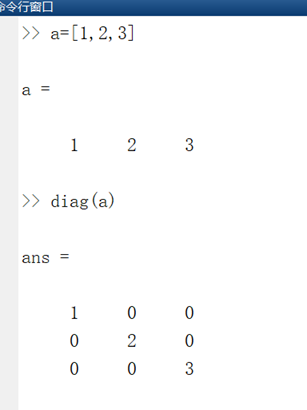5） 随机矩阵
rand(m,n)、rand(m)生成的元素为0—1的随机数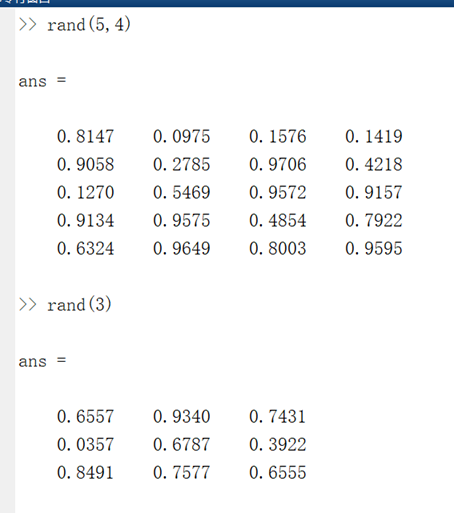randn(m,n)、randn(m)
生成正态分布随机矩阵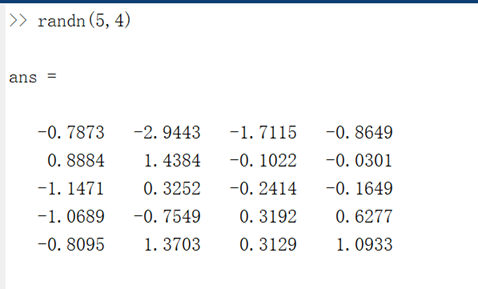展开全文matlab
• ## matlab结构矩阵

千次阅读 2022-01-09 11:34:11
matlab结构矩阵单元创建相关函数总结 单元 结构矩阵中的每一个元素内部还包含不同的成员，即其中数据类型不同，但是逻辑相关。以学生举例： 其中包含学号，姓名，性别； 创建 与C中的创建结构体相似： a(1).x1=10; a...

# 单元

结构矩阵中的每一个元素内部还包含不同的成员，即其中数据类型不同，但是逻辑相关。以学生举例：
其中包含学号，姓名，性别；

# 创建

与C中的创建结构体相似：

a(1).x1=10;
a(1).x2='liu';
a(1).x3=[1,3;3,3];

a(2).x1=20;
a(2).x2='lu';
a(2).x3=[2,2;3,4];

a(3).x1=30;
a(3).x2='u';
a(3).x3=[3,2;3,4]；


在matlab工作区中可以看到如下的结构矩阵：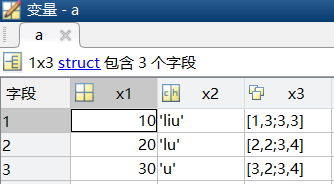# 相关函数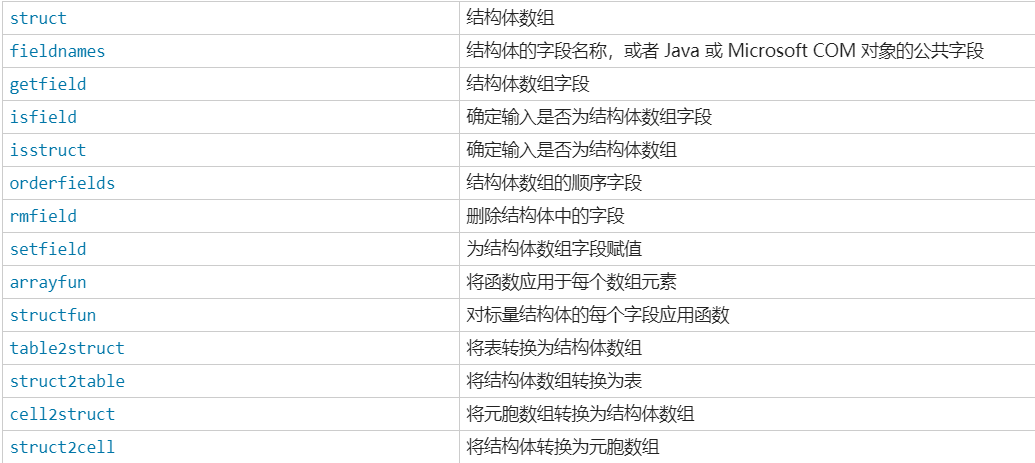struct创建

s = struct
s = struct(field,value)
s = struct(field1,value1,…,fieldN,valueN)
s = struct([])
s = struct(obj)

getfield获取

f=a.(‘x1’)与value = getfield(a, ‘x1’)所得结果相同

rmfield删除

s = rmfield(s,field)
从结构体数组 s 中删除指定的一个或多个字段。使用字符向量元胞数组或字符串数组指定多个字段。s 的维度保持不变。

删除单个字段：

s.a = 1;
s.b = 2;
s.c = 3;
field = 'b';
s = rmfield(s,field)


工作区如下已经没有b字段：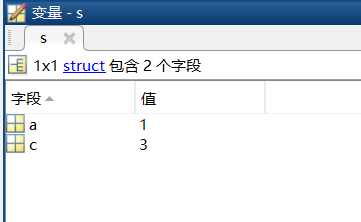删除多个字段：

s.a = 1;
s.b = 2;
s.c = 3;
fields = {'a','b'};
s = rmfield(s,fields)



工作区如下已经没有a,b字段：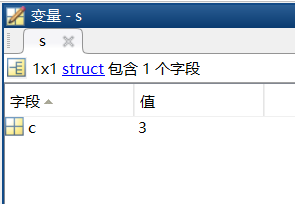setfield修改

s.a = 1;
s.b = 2;
s.c = 3;
s=setfield(s,b,4)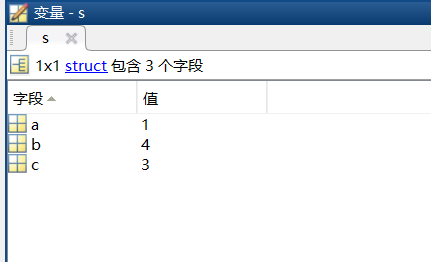# 总结

展开全文matlab
• matlab的使用过程中经常会需要用到矩阵，有时想要创建一些数据比较多的矩阵，对矩阵的内容要求可能并不高或者要创建一些特殊矩阵，下面将提供一些matlab内部提供的一些快速创建矩阵的方法。 1 创建单位矩阵 eye(n)...

在matlab的使用过程中经常会需要用到矩阵，有时想要创建一些数据比较多的矩阵，对矩阵的内容要求可能并不高或者要创建一些特殊矩阵，下面将提供一些matlab内部提供的一些快速创建矩阵的方法。

### 1 创建单位矩阵

eye(n):创建n×n的单位矩阵。
eye(m,n):创建m×n的单位矩阵。
如，eye(3,2)得到的结果为：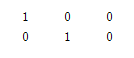### 2 创建全为1的矩阵

ones(n):创建n×n的全为1的矩阵。
ones(m,n):创建m×n的全为1的矩阵。

### 3 创建全为0的矩阵

zeros(n):创建n×n的全为0的矩阵。
zeros(m,n):创建m×n的全为0的矩阵。

### 4 创建随机矩阵

rand(n):创建[0,1]区间内n×n的随机矩阵。
rand(m,n):创建[0,1]区间内m×n的随机矩阵。

### 5 创建对角阵

diag(v):创建以向量v中元素为对角的对角矩阵。
如，diag([1 2 3])得到的结果为：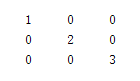### 6 创建希尔伯特（Hilbert）矩阵

hilb(n):创建n×n的Hilbert矩阵。
Hilbert矩阵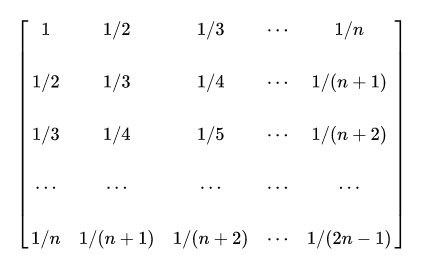### 7 创建魔方矩阵

magic(n):创建n阶魔方矩阵。
魔方矩阵：每行、每列、对角线上的和都相等的矩阵。

以上内容查自《MATLAB2020从入门到精通》与百度百科

展开全文• 诚教:matlab中取矩阵的...如果是取第1,3,5行和第1,4,5列相交的数据为新矩阵,可以表示为：C=A([1,3matlab矩阵建立A=zeros(1,24);B=ones(1,25);A=B(2:25)如此即可,冒号的意思是从第2个元素到第25个.matlab找出矩...
• ## MATLAB 稀疏矩阵

千次阅读 2022-01-07 23:35:54
MATLAB 稀疏矩阵 %零元素个数远远多于非零元素的个数的矩阵 %矩阵的存储方式 %稀疏存储方式：只存储矩阵的非零元素的值及其位置，即行号和列号。 %A=sparse(S):将矩阵S转化为稀疏存储方式的矩阵A。 %S=full(A):将...matlab
•matlab
• 创建一个按行填充1-64的8×8矩阵的最快方法是什么.帮助文档说我甚至应该能够用数组填充矩阵,但我似乎无法使其工作.我被告知它可以比我做得更容易,但我没有看到它完成.这是我正在寻找的想法……v26 =1 2 3 4 5 6 7 89...
• 编写脚本文件、建立一个nxn的螺旋矩阵并输出，其中元素为1到nxn的自然数。从左上角开始，依次沿顺时钟方向由外及里存放。n的值从键盘输入。参考如下图片。 clc; clear; n=input('输入方阵行数 n='); result=zeros(n...matlab
• 1.矩阵的输入 >> A=[] A = [] >> B=[6 5 3] B = 6 5 3 >> C=B' C = 6 5 3 2.调用矩阵 >> A=[1 2 3 4 5] A = 1 2 3 4 5 >> a1=A(1)%访问数组第一个元素 a1 = ...matlab
• 1、特殊矩阵的调用格式 例 >> a = zeros(2) a = 0 0 0 0 >> b = zeros(2,3) b = 0 0 0 0 0 0 >> c = zeros(size(reshape(b,3,2))) c = 0 0 0 0 0 0 分析：①产生两位随机整数[10...matlab
• 1.直接输入创建矩阵： （1）.“[]”作为首尾； （2）.“,”或空格隔开； （3）.";"或Enter键分隔。 ...2.用矩阵函数生成矩阵MATLAB提供了大量的函数，来创建一些特殊的矩阵。 eye(3) zeros(3,4) ...matlab
• 矩阵定义 ...利用MATLAB函数创建矩阵 基本矩阵函数如下： (1) ones()函数：产生全为1的矩阵，ones(n)：产生nn维的全1矩阵，ones(m,n)：产生mn维的全1矩阵； (2) zeros()函数：产生全为0的矩阵； (3) ra
• MATLAB中创建矩阵的方法一般有3种：第一种是直接输入矩阵元素；第二种是通过调用函数生成特殊矩阵；第三种是利用M文件创建矩阵。 1.元素直输法创建矩阵MATLAB中，创建一个矩阵最简单的方法是使用矩阵构造符号...MATLAB 创建矩阵
• ## matlab循环矩阵

千次阅读 2021-04-20 01:17:59
参考：...clear; h = [10 9 8 7 6 5 4 3 2 1];size=length(h);t=zeros(1,size);t(1)=h(1);t(1,2:size)=h(size:-1:2);H=toeplitz(h,t)这个也ok：clc;clear;h = [10 9...
• 如何在MATLAB中创建矩阵，将矩阵随机分组，并随机取组赋值 欢迎使用Markdown编辑器 你好！ 这是你第一次使用 Markdown编辑器 所展示的欢迎页。如果你想学习如何使用Markdown编辑器, 可以仔细阅读这篇文章，了解一下...matlab
• MATLAB矩阵有两种存储方式，完全存储方式和稀疏存储方式 1.完全存储方式将矩阵的全部元素按列存储，矩阵中的全部零元素也存储到矩阵中。 2.稀疏存储方式仅存储矩阵所有的非零元素的值及其位置，即行号和列号，显然...
• ## MATLAB矩阵的表示

千次阅读 2022-05-11 23:51:41
利用直接输入法建立矩阵 建立实矩阵 建立复矩阵 利用已建好的矩阵建立更大的矩阵 大矩阵由已建好的小矩阵拼接而成 用实部矩阵和虚部矩阵构成复数矩阵 冒号表达式 矩阵元素的引用 矩阵元素的引用方式 矩阵...matlab
• MATLAB矩阵的建立与访问 数值数据的输出格式（format） 一、 MATLAB 函数的调用格式 常用函数的应用 二、MATLAB矩阵表示及矩阵元素的引用 (1) 利用直接输入法建立矩阵 (2) 利用已建好的矩阵建立更大的矩阵 (3) 用...matlab python
• 1、普通矩阵建立不管是任何矩阵(向量)，都可以直接按照行方式输入每个元素：同一行的元素用逗号或者用空格符来分割，且空格个数不限；不同的行用分号分割。所有的元素都处于一方括号[]内。比如：>>Matrix_2D...matlab矩阵访问
• ## Matlab矩阵运算

千次阅读 2021-04-22 03:29:59
•   Matlab结构矩阵用法类似C语言结构体，也可定义一组变量，类型可以随意，并且不用声明变量类型。其基本形式为：            结构矩阵元素.成员名 = 表达式 例如： a(1).x1 = 10; a(1).x2 = 'liu'...matlab
• ## MATLAB矩阵运算

千次阅读 2022-04-23 20:31:11
MATLAB矩阵作为数据操作的基本单位，这使得矩阵运算变得非常简捷、方便、高效。矩阵是由m×n个数av (i=1,2,…,m; j = 1,2,…,n）排成的m行n列数表，记成： 若m=n，则该矩阵为n阶矩阵(n阶方阵）。 矩阵创建 ...matlab
• ## matlab矩阵操作

千次阅读 2022-01-17 14:27:38
矩阵matlab中最基本的数据对象，matlab的大部分运算和命令都是在矩阵运算的意义下进行的。 一、矩阵建立 直接输入法： 将矩阵的元素用中括号括起来，按矩阵行的顺序输入各元素，同一行的各元素之间用逗号或...matlab
• Jim Thorpe was a Native American. He was born in 1888 in an Indian Territory( 印第安人 保护区)that is now Oklahoma. Like most Native American children then, he liked to fish, hunt, swim, and play ...
• ## MATLAB结构矩阵

千次阅读 2020-07-04 11:34:25
• 第2章 MATLAB矩阵及其运算 2.1 变量和数据操作 2.2 MATLAB矩阵 2.3 MATLAB运算 2.4 矩阵分析 2.5 矩阵的超越函数 2.6 字符串 2.7 结构数据和单元数据 2.8 稀疏矩阵 2.1 变量和数据操作 2.1.1 变量与赋值 1....
• Matlab操作矩阵的相关方法下面这篇文章主要是对吴恩达老师机器学习中matlab操作的一个整理和归纳一、基本操作1.生成矩阵(ones、zeros)A = [1 2;3 4;5 6] #生成3行4列的矩阵B = [1 2 3] #B就是一个行向量C = [1;2;3] ...
• ## matlab矩阵创建

千次阅读 2020-10-22 22:43:41
matlab矩阵的创建： 1.矩阵的创建方法 1.1直接输入法： 以【】作为矩阵开始和结束 矩阵行与行之间用‘；’隔开 同一行不同元素用逗号或者空格符来分开 矩阵元素可以采用表达式 ex: M=[1 2 3 4 5 6;sqrt(25),10-5,0...matlab...